### Wave Reflection at an Impedance Discontinuity

 Wave Reflection at an Impedance DiscontinuityThe impedance for mechanical waves is defined as the ratio between the force wave and velocity wave and in general takes the for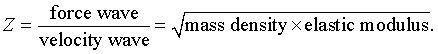For transverse waves in a string with linear mass density(kg/m) and tension T (N), the impedance is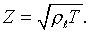If two strings with different mass densities are connected with a common tension, the impedance discontinuity at the joint causes wave reflection. For an incident displacement wave of unit amplitude in string 1, the reflected wave is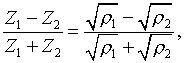and transmitted wave is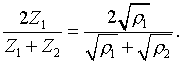The first animation shows reflection and transmission of incident pulse wave of unit amplitude when the mass density ratio is 4, Z1/Z2 = 1/2. The reflected wave is negative and its peak is -1/3. The transmitted wave, which propagates slower, has an amplitude of 1 - 1/3 = +2/3, as expected from the formulae. The second animation shows the case Z1/Z2 = 2, i.e., the incident wave is in a heavier string. There is no sign reversal in the reflected wave in this case. The transmitted wave has an amplitude larger than the incident wave. This does not mean amplification in wave energy. (Why not?)The third animation shows reflection at a fixed end (Z2 = infinity) and fourth animation shows reflection at a free end (Z2 = 0). Reflection of pulse wave at an impedenace discontinuity when Z_1/Z_2 = 0.5.> with(plots): animate((exp(-(x-t)^2)-1/3*exp(-(x+t)^2))*Heaviside(-x)+2/3*exp(-4*(x-.5*t)^2)*Heaviside(x),x=-10..10,t=-10..10,frames=50,color=red,numpoints=200);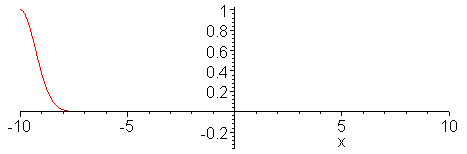When Z_1/Z_2 = 2.0.> with(plots): animate((exp(-(x-t)^2)+1/3*exp(-(x+t)^2))*Heaviside(-x)+4/3*exp(-.25*(x-2*t)^2)*Heaviside(x),x=-10..20,t=-10..10,frames=50,color=red,numpoints=200);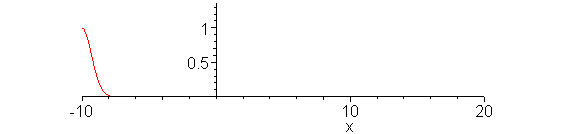When Z_2 = infinity. (Fixed end)> with(plots): animate((exp(-(x-t)^2)-exp(-(x+t)^2)),x=-10..0,t=-10..10,frames=50,color=red,numpoints=200);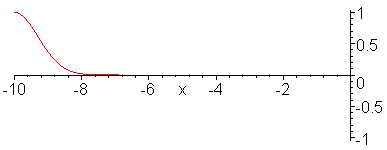When Z_2 = 0. (Free end)> animate((exp(-(x-t)^2)+exp(-(x+t)^2)),x=-10..0,t=-10..10,frames=50,color=red,numpoints=200);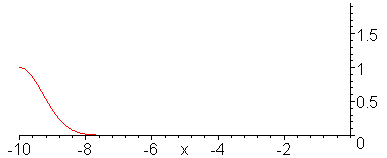Ċ
Jason Lin,
2018年9月6日 凌晨12:44